# * J Determine the slope at A and displacement at point C. El is constant. Use...

*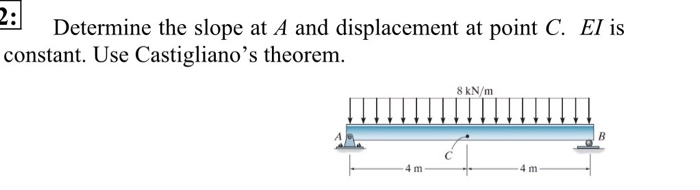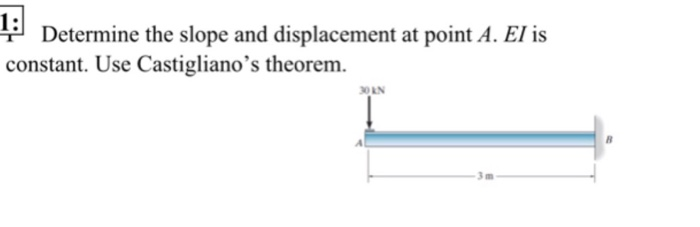J Determine the slope at A and displacement at point C. El is constant. Use Castigliano's theorem. 8 kN/m - 4 m
3: Determine the displacement at point C. EI is constant. Use Castigliano's theorem. (Page 1 of 1
Determine the slope and displacement at point A. El is constant. Use Castigliano's theorem. ON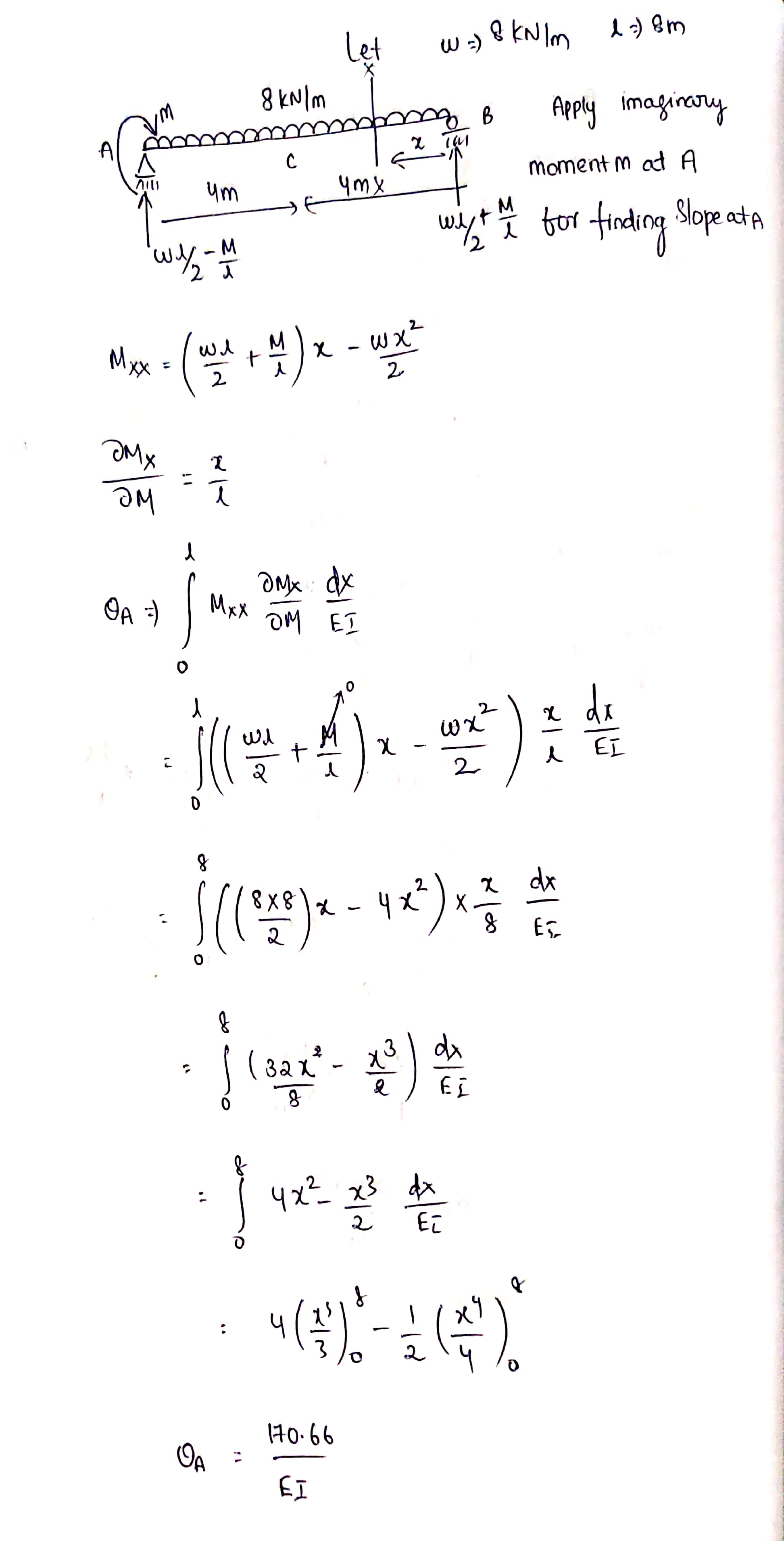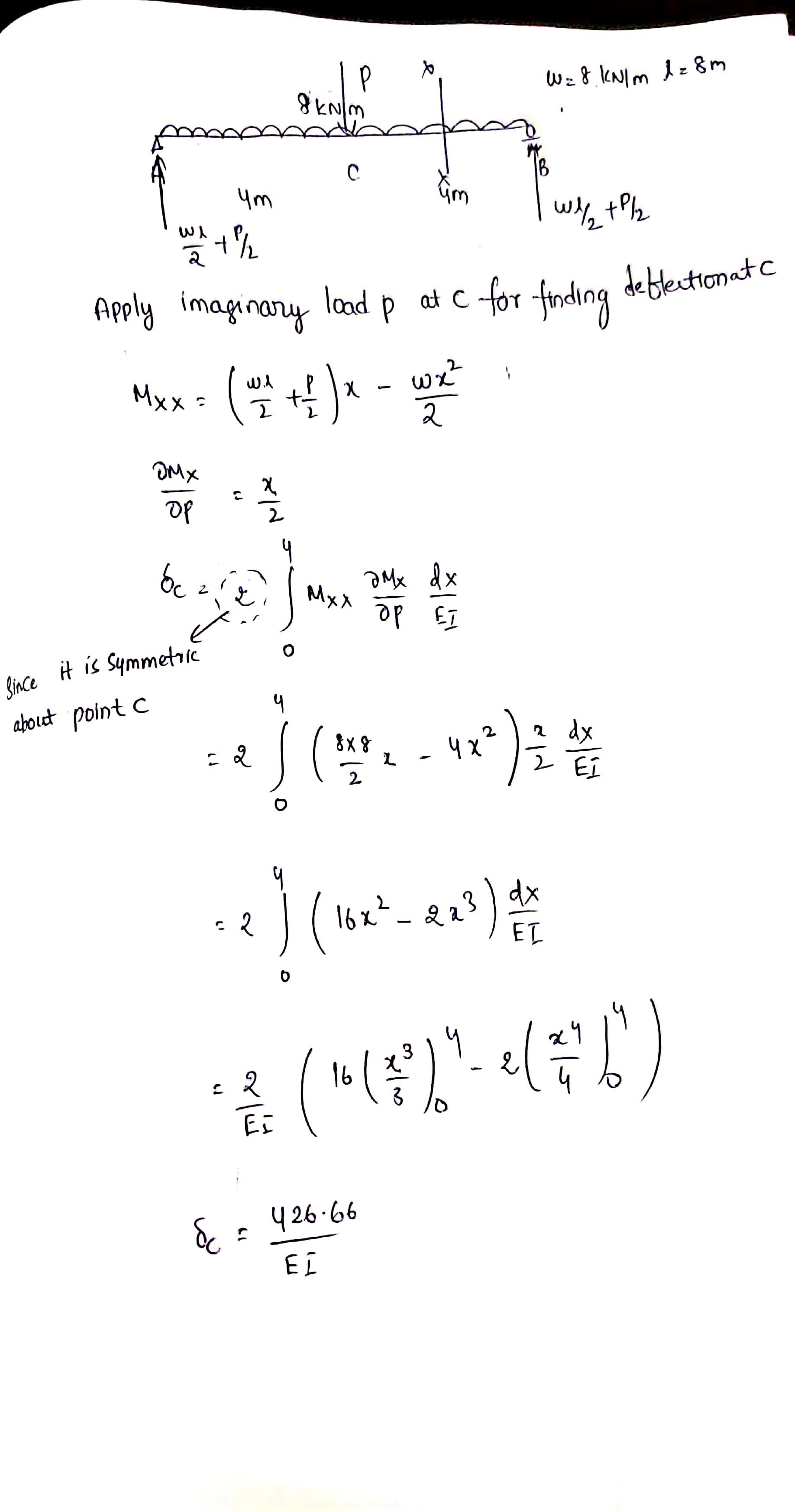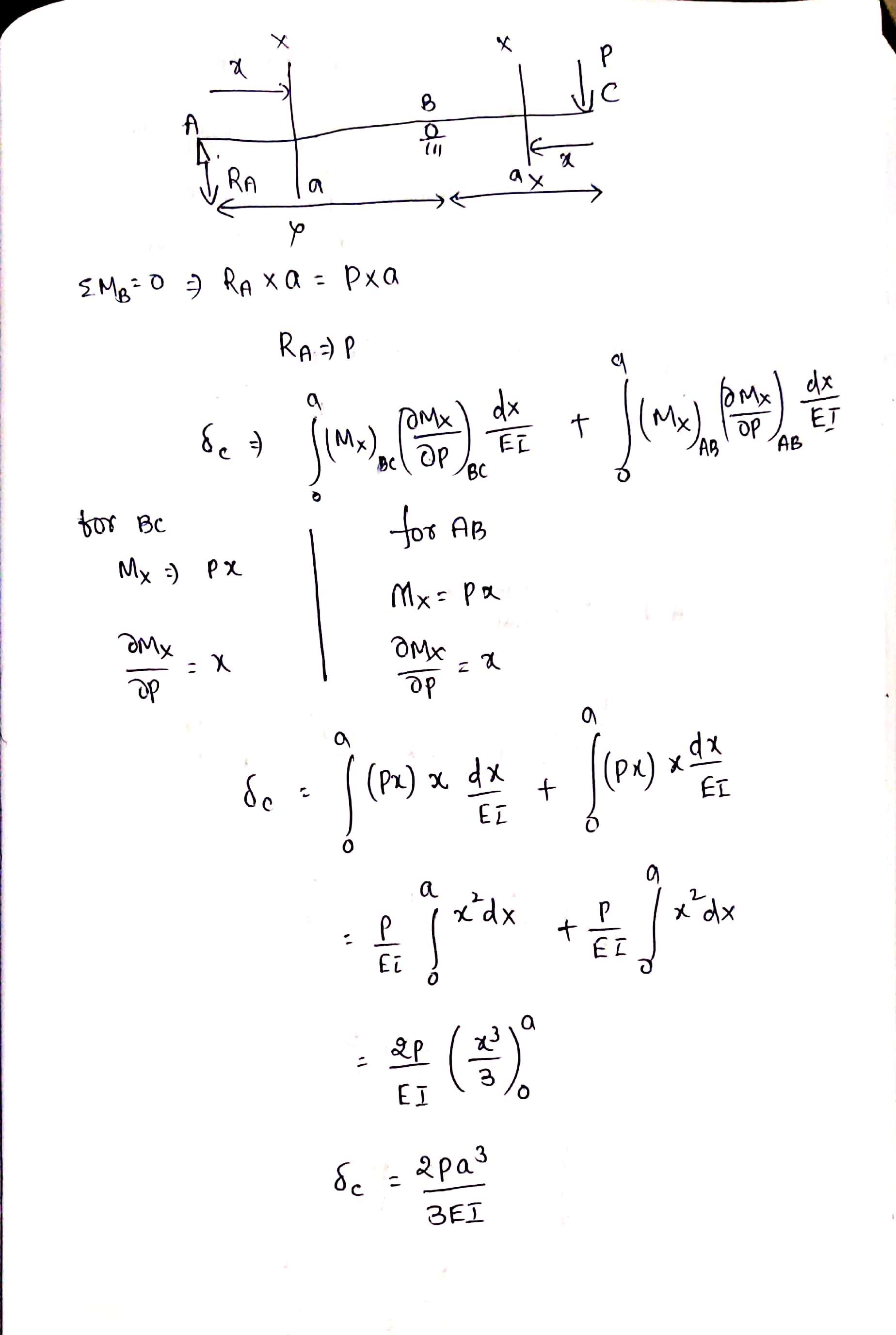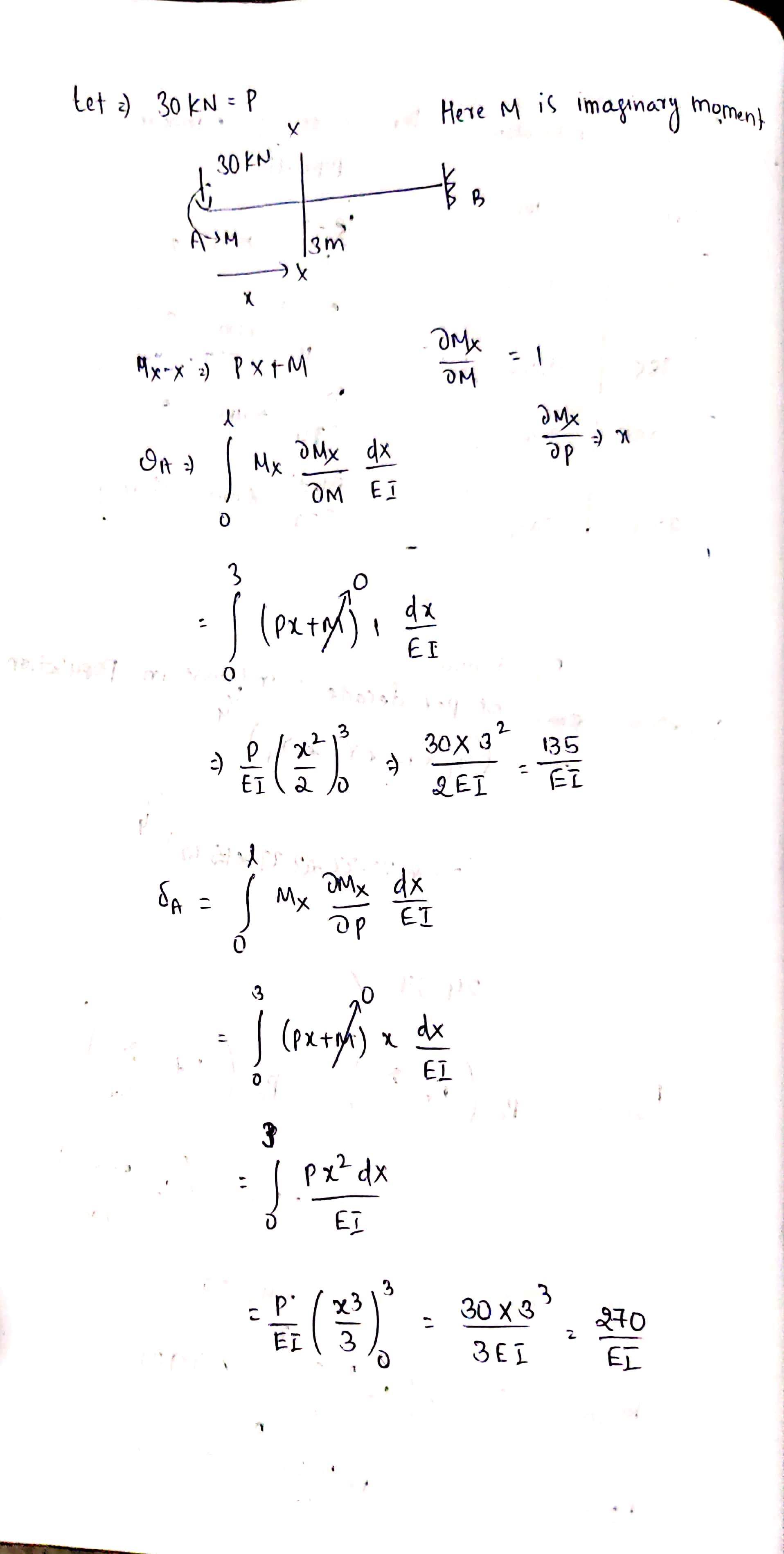#### Earn Coin

Coins can be redeemed for fabulous gifts.

Similar Homework Help Questions
• ### 8-55 : Determine the vertical displacement of point C. EI is constant. Use the method of...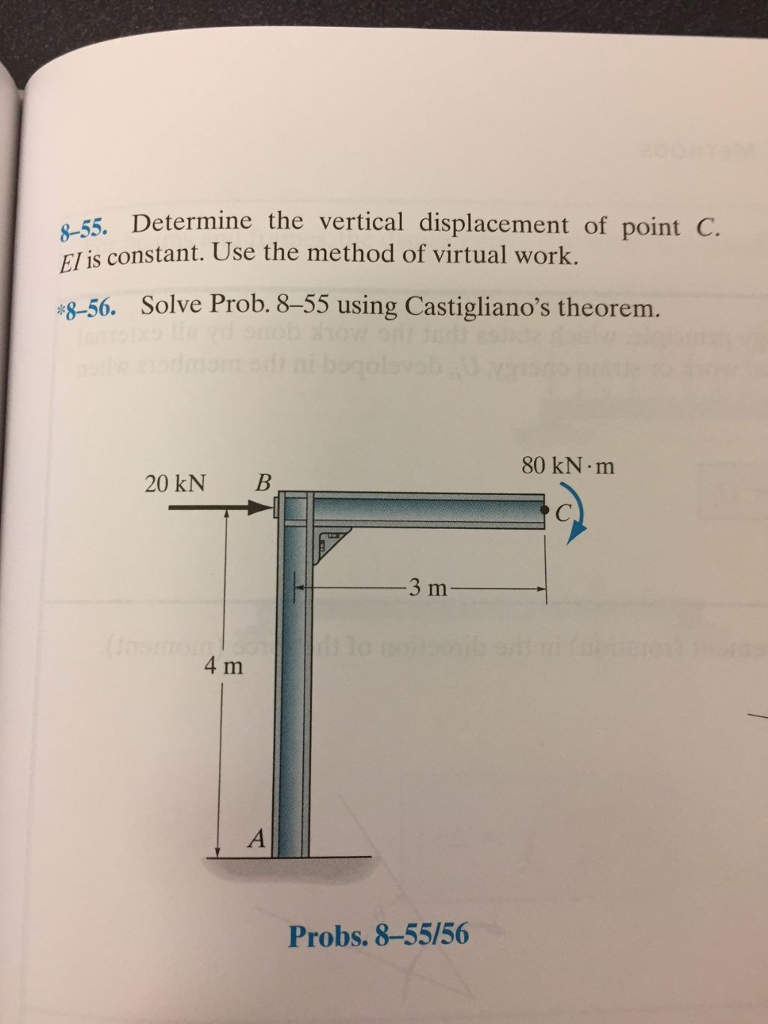8-55 : Determine the vertical displacement of point C. EI is constant. Use the method of virtual work. -55. Determine the vertical displacement of point C. El is constant. Use the method of virtual work. \$-56. Solve Prob. 8-55 using Castigliano's theorem. 80 kN m 20 kN B 4 m Probs. 8-55/56

• ### Determine the slope and displacement at point A. Assume C is pinned. (Answer encircled letter only)...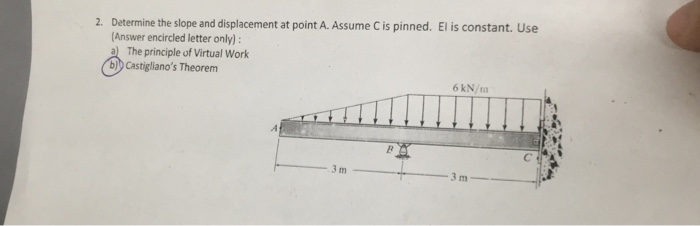Determine the slope and displacement at point A. Assume C is pinned. (Answer encircled letter only) a The principle of Virtual Work b)b Castigliano's Theorem El is constant. Use 2. 6 kN/m 3 m

• ### Review Part B Consider the beam shown in (Figure 1). El is constant. Suppose that EI...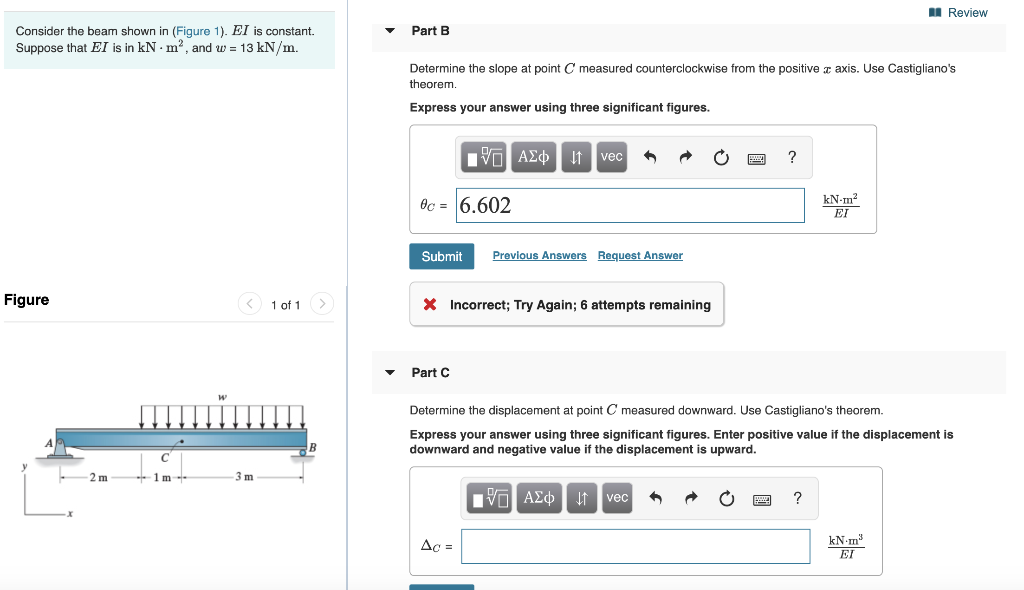Review Part B Consider the beam shown in (Figure 1). El is constant. Suppose that EI is in kNm", and w = 13 kN/m. Determine the slope at point C measured counterclockwise from the positive 3 axis. Use Castigliano's theorem. Express your answer using three significant figures. PO ASC u veca O ? 06 = 6.602 Submit Previous Answers Request Answer Figure < 1 of 1 X Incorrect; Try Again; 6 attempts remaining Part Determine the displacement at point C...

• ### Determine the vertical displacement of point C. El is constant. Use the method of virtual work....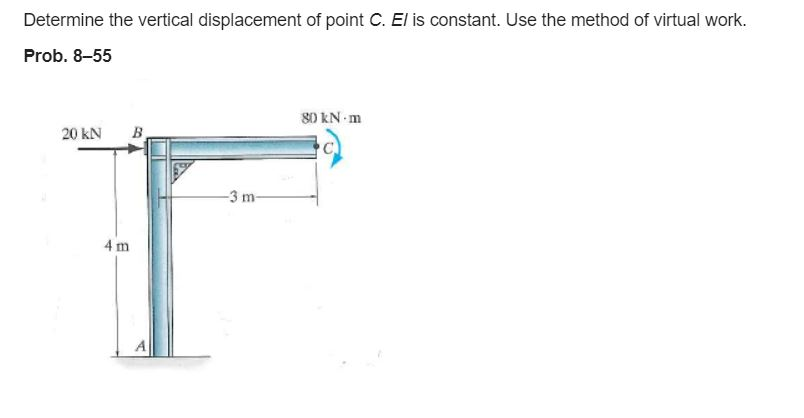Determine the vertical displacement of point C. El is constant. Use the method of virtual work. Prob. 8-55 80 KN.m 20 KN B -3 m 4 m

• ### Determine the slope and displacement at point C. El is constant. Assume A is a pin....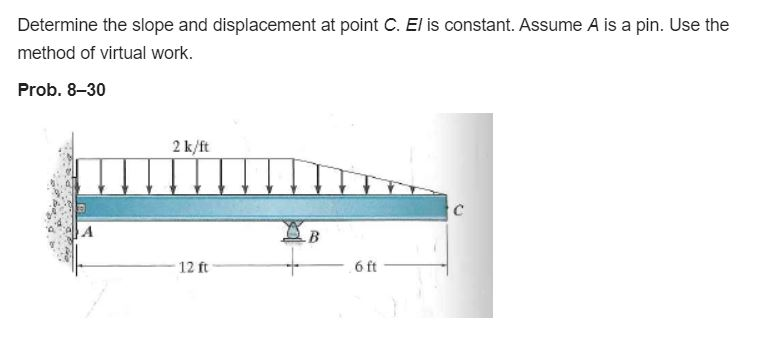Determine the slope and displacement at point C. El is constant. Assume A is a pin. Use the method of virtual work. Prob. 8-30 2 k/ft с А 12 ft 6 ft

• ### 5-Use moment area method. Determine the vertical displacement of point D. Use moment area method. A is fixed, B is a hinge and C is a roller. Note that El is not constant. 2KN 2 KN/m EI EI D 2E...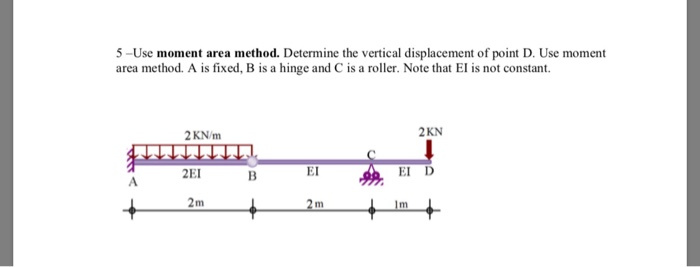5-Use moment area method. Determine the vertical displacement of point D. Use moment area method. A is fixed, B is a hinge and C is a roller. Note that El is not constant. 2KN 2 KN/m EI EI D 2EI 1m 5-Use moment area method. Determine the vertical displacement of point D. Use moment area method. A is fixed, B is a hinge and C is a roller. Note that El is not constant. 2KN 2 KN/m EI EI D...

• ### Q.3: Determine the slope and displacement at point B. El-70000 Nm2 is constant. Use the method...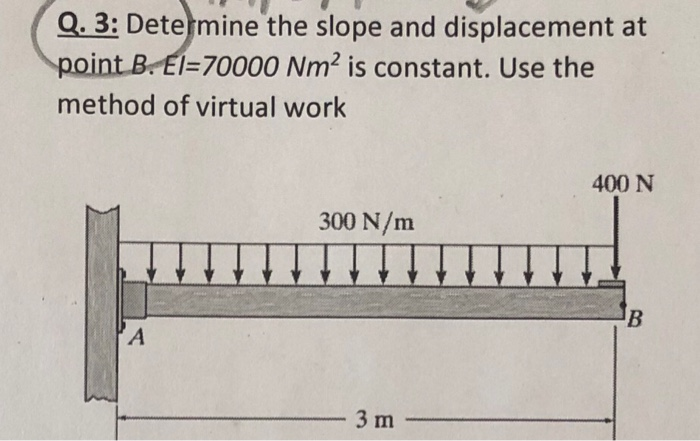Q.3: Determine the slope and displacement at point B. El-70000 Nm2 is constant. Use the method of virtual work 400 N 300 N/m B A 3 m 1

• ### Determine the slope and displacement at point C. Use double integration method. Note : EI is...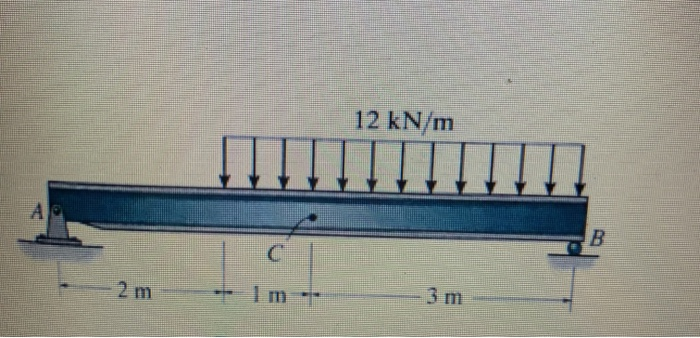Determine the slope and displacement at point C. Use double integration method. Note : EI is constant Please, check the answer: 12 kN/m B 2 m 4.67 kN.m E \$21. & 1525 kNm

• ### 1. Determine the slope and displacement at point C. El is constant. Assume A is a...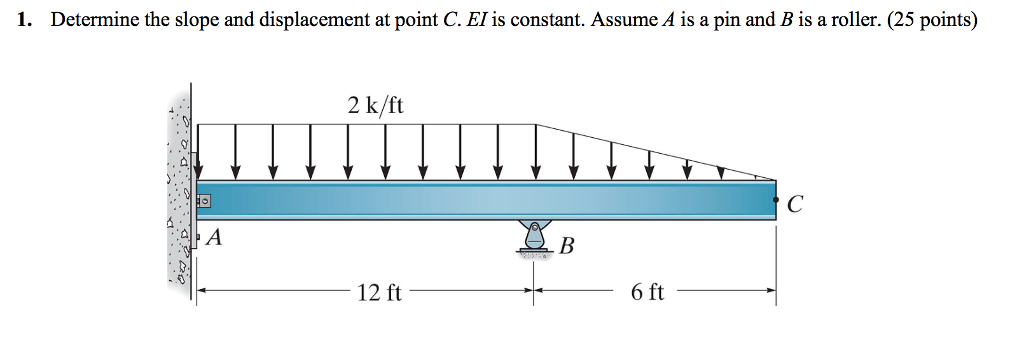1. Determine the slope and displacement at point C. El is constant. Assume A is a pin and B is a roller. (25 points) 2 k/ft 12 ft 6 ft

• ### Determine the displacement at point D. Use the principle of virtual work. El is constant. 60...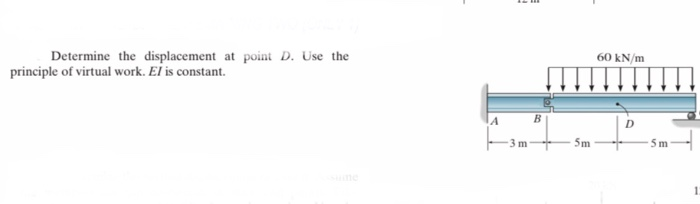Determine the displacement at point D. Use the principle of virtual work. El is constant. 60 kN/m А B 3 m 5m Sm